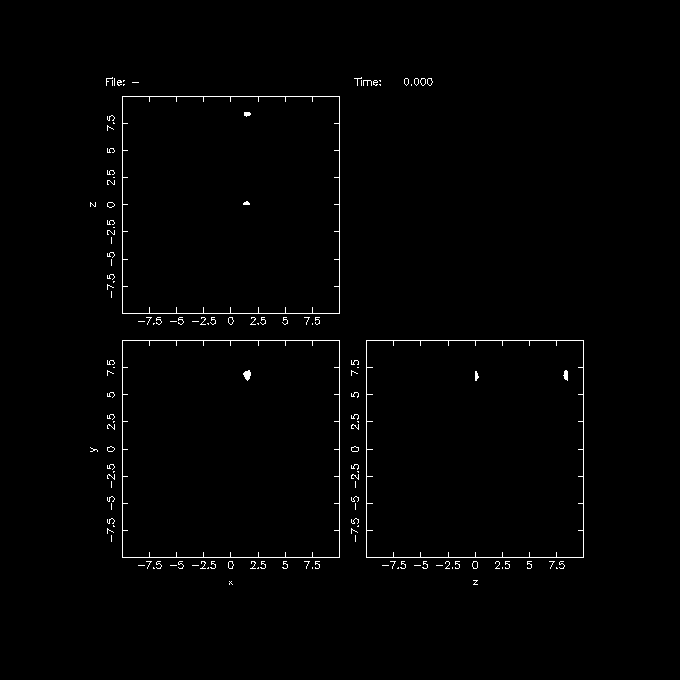# 18. RST reminders¶

## 18.1. Lists¶

• Bullet are made like this

• A link to the NEMO repo, or github pages.

• Links to the man pages: github or local

• Links to some old examples: github or local

• Links to some Restructuredtext RST guides:
• Point levels must be consistent
• Sub-bullets
• Sub-sub-bullets

• Lists

Term

Definition for term

Term2

Definition for term 2

List of Things

item1 - these are ‘field lists’ not bulleted lists item2 item 3

Something

single item

Someitem

single item

## 18.2. Code blocks¶

There are three equivalents: code, sourcecode, and code-block.

# some python code
import os
print(help(os))
if True:
print("yes")
else:
print("no")

# Equivalent

# Equivalent

# some bash code
if [ -e /tmp ]; then
echo "You have /tmp"
fi


## 18.3. Images¶

Here is an image …from the eagle example.

## 18.4. Tables¶

Time

Number

Value

12:00

42

2

23:00

23

4

### 18.4.1. Math¶

Some example math, taken from latex

$\ddot{ {\bf r}}_i \, = \, -G \sum_{j=1;\, j \not = \,i}^N {m_j \,({\bf r}_i - {\bf r}_j) \over {(r_{ij}^2 + \epsilon^2)^{3/2}$

or inline :math: c^2 = a^2 + b^2